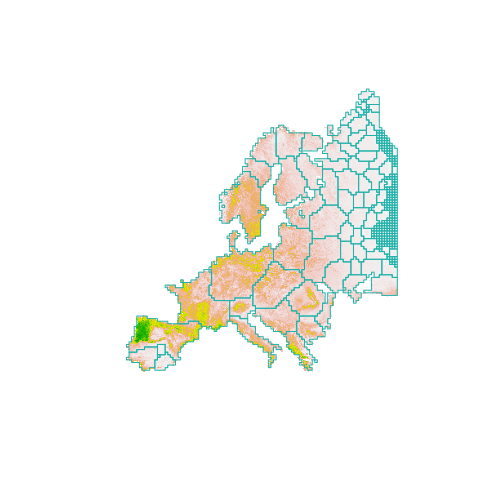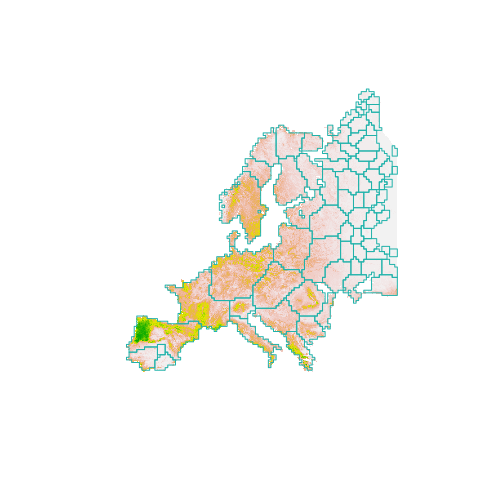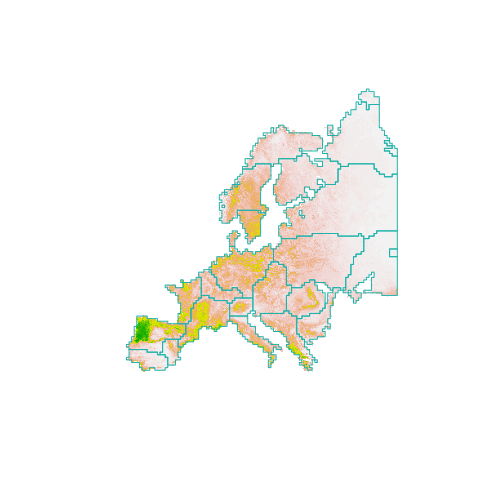# introduction-to-habCluster

You can install the development version of habCluster from Cran with:

install.packages("habCluster")

or from GitHub with:

# install.packages("devtools")
devtools::install_github("qiangxyz/habCluster")

## Example

This is a basic example which shows you how to find the cluster of lands:

Read in habitat suitability index (HSI) data of wolf in Europe. The HSI values of the cells in the raster indicate how smoothly the wolfs can moved in the cells, and can be used to represent the connection between cells as habitat. The original value of HSI was 0 - 1.0 (float), yet was transformed to integers from 0 - 100 for reducing the file size.

hsi.file = system.file("extdata","wolf3_int.tif",package="habCluster")
# rescale raster value to 0 - 1
wolf = wolf / 100

Find habitat cluster using Leiden Algorithm. Raster for habitat suitability will be resampled to 40 km (40000 m), to reduce calculation amount. Set cluster_resolution_parameter to 0.02 to control the cluster size (only for method of cluster_leiden). Note that the parameter of cellsize controls the spatial scale analysis is performed, while the parameter of rp is used to control cluster size.

clst = cluster(wolf, method = cluster_leiden, cellsize = 40000, resolution_parameter = 0.0002, silent = FALSE)
#>
#> resampling...
#> extracting edges...
#> create graph...
#> finding clusters...
#> preparing results...

We can also embed plots, for example:

image(wolf, col = terrain.colors(100,rev = T), asp = 1)
boundary = clst$boundary plot( boundary$geometry, add=TRUE, asp=1, border = "lightseagreen")Or, we can discard small patches before plotting:

image(wolf, col = terrain.colors(100,rev = T), asp = 1)
boundary$area = as.numeric(st_area(boundary)) boundary = boundary[boundary$area > 40000*40000,]
plot( boundary$geometry, add=TRUE, asp=1, border = "lightseagreen")Leiden algorithm is not greedy, therefore the clusters are somehow random. We can use the Fast Greedy algorithm to avoid random results: clst = cluster(wolf, method = cluster_fast_greedy, cellsize = 40000) image(wolf, col = terrain.colors(100,rev = T), asp = 1) boundary = clst$boundary
plot( boundary\$geometry, add=TRUE, asp=1, border = "lightseagreen")Can also using RasterLayer to manipulate the raster:

# library(raster)
# wolf = raster(hsi.file)
# wolf = wolf / 100
# clst = cluster(wolf, method = cluster_leiden, cellsize = 40000, rp = 0.0002, silent = FALSE)